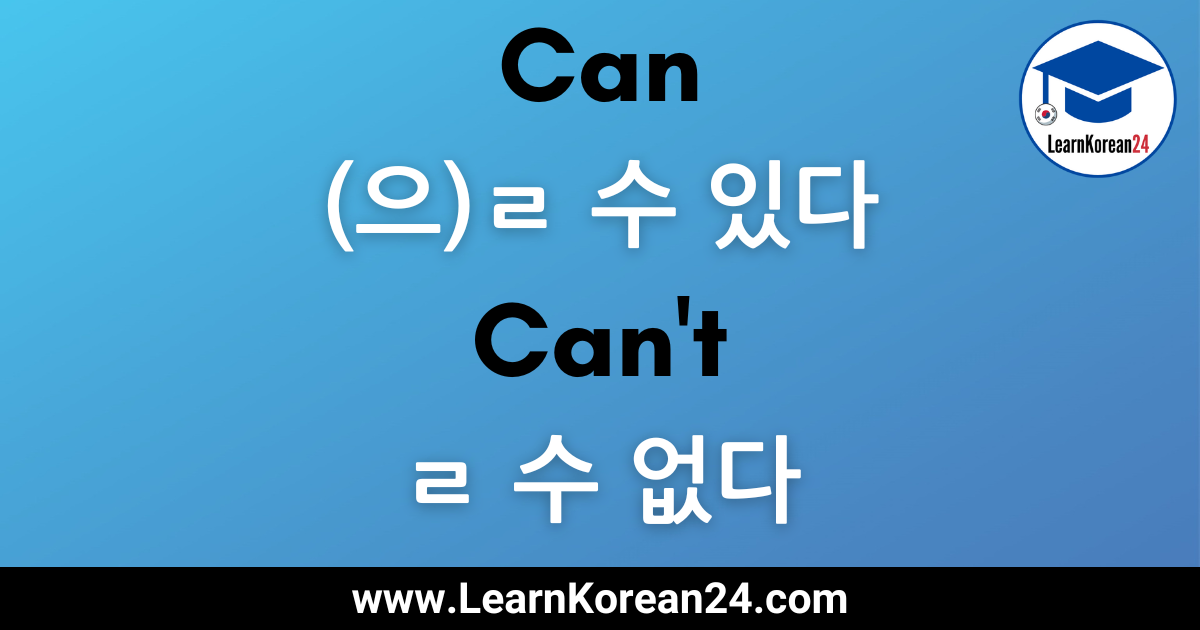# Can And Can’t In KoreanIn this Korean lesson, you will learn how to say can and can’t in Korean. Being able to say that you can do something or can’t do something can be super useful when communicating in Korean. Below, you’ll learn how to say can and can’t in Korean and how to ask and answer questions about ability in Korean.

## ‘Can’ In Korean: -(으)ㄹ 수 있다

To say ‘can’ in Korean you attach –(으)ㄹ 수 있다 to an action verb to indicate that you can do that action. For example, 읽다 (to read) plus (으)ㄹ 수 있다 (can) is 읽을 수 있다 (can read).

### Conjugation

When attaching (으)ㄹ 수 있다 to a verb stem which ends in a consonant, 을 수 있다 is added. Here are some examples:

• 먹다 (to eat) + 을 수 있다 = 먹을 수 있다 (can eat)
• 읽다 (to read) + 을 수 있다 = 읽을 수 있다 (can read)

When attaching (으)ㄹ 수 있다 to a verb stem which ends in a vowel, is dropped and ㄹ 수 있다 is added. Here are some examples:

• 하다 (to do) + ㄹ 수 있다 = 할 수 있다 = (can do)
• 가다 (to go) + ㄹ 수 있다 = 갈 수 있다 = (can go)

Exceptions: When attaching (으)ㄹ 수 있다 to verb stems which end in , it follows a different conjugation rule. This is because all verbs ending with are irregular. When you want to conjugate a irregular verb with an ending which starts with (으)ㄹ, you must drop (으)ㄹ from the ending.

For example, 살다 (to live) + (으)ㄹ 수 있다 = 살 수 있다 (can live). This is because is dropped from the ending. So, it becomes 살다 + 수 있다 which then becomes 살 수 있다.

## ‘Can’t’ In Korean: -(으)ㄹ 수 없다

To say ‘can’t’ in Korean you attach -(으)ㄹ 수 없다 to an action verb to indicate that you can’t do that action. For example, 읽다 (to read) plus –(으)ㄹ 수 없다 (can’t) = 읽을 수 없다 (can’t read).

### Conjugation

When attaching -(으)ㄹ 수 없다 to a verb stem which ends in a consonant, 을 수 없다 is added. Here are some examples:

• 먹다 (to eat) + 을 수 없다 = 먹을 수 없다 (can’t eat)
• 읽다 (to read) + 을 수 없다 = 읽을 수 없다 (can’t read)

When attaching (으)ㄹ 수 없다 to a verb stem which ends in a vowel, is dropped and ㄹ 수 없다 is added. Here are some examples:

• 하다 (to do) + ㄹ 수 없다 = 할 수 없다 = (can’t do)
• 가다 (to go) + ㄹ 수 없다 = 갈 수 없다 = (can’t go)

Exceptions: Again, with verbs that end in , the above rules don’t apply because ㄹ verbs are irregular. As was mentioned above, when you want to conjugate a ㄹ irregular verb with an ending which starts with (으)ㄹ, you must drop (으)ㄹ from the ending. So, when adding (으)ㄹ 수 없다 to a verb which ends in , is dropped and you simply add 수 없다. For example, 살다 (to live) + (으)ㄹ 수 없다 = 살 수 없다 (can’t live).

## ‘Can’t’ In Korean: 못

Another way to say ‘can’t’ in Korean is to use the word [mot]. is more common in spoken language as it is shorter and easier to say. To use to say you cannot do something, you simply add [mot] before a verb. Let’s look at some examples.

갈 수 없다 = can’t go
못 가다 = can’t go

읽을 수 없다 = can’t read